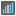• # error - doesn't happen when running python locally

## Question related to missionMedian

I would like to give some feedback about ...

"it returns a type error, saying list indices must be integers not floats.  well they are..."

From: http://www.checkio.org/mission/median/solve/

HTTP\_USER\_AGENT:

Mozilla/5.0 (X11; Ubuntu; Linux x86_64; rv:30.0) Gecko/20100101 Firefox/30.0

My Code:

def checkio(data):
data.sort()
if len(data) % 2 == 0:
median = ((data[len(data)/2-1] + data[len(data)/2]) / 2.0)
else:
median = data[(((len(data) - 1)) / 2)]
return median

#These "asserts" using only for self-checking and not necessary for auto-testing
if __name__ == '__main__':
assert checkio([1, 2, 3, 4, 5]) == 3, "Sorted list"
assert checkio([3, 1, 2, 5, 3]) == 3, "Not sorted list"
assert checkio([1, 300, 2, 200, 1]) == 2, "It's not an average"
assert checkio([3, 6, 20, 99, 10, 15]) == 12.5, "Even length"
print("Start the long test")
assert checkio(list(range(1000000))) == 499999.5, "Long."
print("The local tests are done.")